### Some advanced algorhytms

#### Gauss method for solving systems of linear equations

It's easy to solve such a systems using matrix algebra (evaluating determinants etc.) but sometimes it's more convenient to use Gauss method. The idea is to exclude variables on each step by multiplying the whole equation on some coefficient and then by subtracting this equation from next equation in the system so that the coefficient near some variable to become zero. The resulting system after this operations looks like this:

a*x + b*y + c*z + d = 0
e*x + f*y             + g = 0
i*x                        + j = 0

Such a system is much more convenient to solve than the initial. Here is the program source for Pascal implementing such a method Gauss.pas.

#### Recurrent Polish Notation

The most simple system of writing mathematical expressions for computer is recurrent polish notation. For example, the expression "(a + b)*(c - d)/sin(e)" is written as "a b + c d - * e sin /". You do not need parenthesis in this system and all the expressions can be simply evaluated using stack structure. Just push all the variables in stack, and when meet the function or operation, pop them out, perform the operation and push the result in the stack back. At the end, pop the result from the stack (if the expression is written correctly, it will be the only element in the stack).

Translating traditional forms of recording of mathematical expressions into RPN is a bit more complicated task. It is also solved using stack, but now it is the stack of mathematical operations. The hierarchy of mathematical operations is this:

1. Single functions such as sin, cos etc. and parenthesis
2. Multiplying and division
3. Adding and subtracting

The idea of method is like this:

• If you meet variable in the initial string, write it directly in the RPN string
• If you meet mathematical operation sign, then pop all operations which have higher priority then push it into the stack
• If you meet opening parenthesis, push it into the stack
• If you meet closing parenthesis, pop everything until you meet opening parenthesis Parenthesis' must not be written in the RPN string.
• When all initial string is already scanned, pop all operations into RPN string

Here is Delphi unit, implementing such a method Calculator.pas.

#### Approximate methods

almost all approximate methods are based on Tailor's series: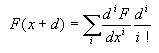Note that sometimes this works only for margined d, and sometimes the best approximation is not achieved with increase of number of iterations. To find approximation with required error, just compare each next part of the sum with this error

Here is a sample code on evaluating Tailor's series Tailorrw.pas

Note the method to solve systems of any equations. Let the system to be written in the form fi(x1,x2,...,xn)=0, i=1..n. Then, using linear approximation, let's find each next k's approximation by solving such a system of linear equations relative to xjk: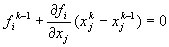In case of one-dimensional function it has such a simple meaning: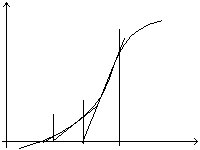#### Some useful equations

•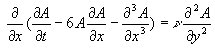- To model 2D-waves on liquid surfaces (resisting forces is also taken into account).
•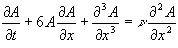- To model one-dimensional solitons on liquid surfaces
•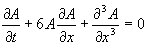- To model one-dimensional solitons in plasma, on liquid surfaces etc.
•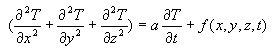- To model non-stable temperature fields
•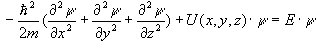- Schredinger's equation to evaluate distribution of possibilities in quantum system.
•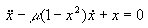- Van-der-Pohl equation for modelling radiowave generator

#### Golography and interference modelling

Both interference and golography as the application of interference require only adding big number of harmonic fields (all with one defined frequency, but different phases) and evaluating the resulting amplitude as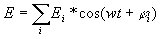. The result is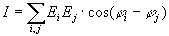Here is the sample program source for Delphi Golography.zip

#### Charged particles motion modelling.

This is only the solving of Newton's (or Einstein's) equation of motion, in the resulting field of all particles. So here is the sample program source for Delphi Motion.zip

#### Bitmap file compression using two-dimensional Fourier analysis

Like one-dimensional case, in two-dimensional space Fourier analysis is implemented by such formulae: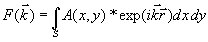Note now we are working with wave vectors k and the phase is evaluated as f=k*r, where r = {x, y}. So the initial bitmap is compressed into the set of wave vectors. Note the quality is really excellent ;)), but remember this is only an experimental program. Here is the sample program source for Delphi Compression.pas.

#### Advanced "LIFE" games

In this version of popular game you have "mammals" (positive numbers) and "predators" (negative numbers), it is possible to "feed" them, note "mammals" and "predators" eat "postive" and "negative" food. Use keys "up","down","left","right" to move the current postion, use +/- keys (withou Shift) to set new postive/negative "beings", use "0" and "9" keys to set food areas (positive/negative). Press Esc to start evolution!
Here is also the "Life" games in 3D-space and on the surface of torus - note that it is a topologically merged continuum!
Here is the sample program source for Pascal Life.pas
The other samples is for Delphi and OpenGL under Delphi: Life3D.zip LifeTor.zip
Compiled programs: LifeTor.exe

#### Less square method

Less square method is a simple and quick method to obtain unknown parameters in functional dependances and to estimate uncertainity of this parameters. Let the expected dependance to be
y = f(x), and we have obtained the set of measured values (xi, f(xi)). Then the resulting error can be estimated as a sum of squared deviations from theoretically expected dependance: (xi - x0)2, where x0 is an average value of x. Let's minimize this error. Thus we have to set the first derivative of this sum with respect to all the parameters to zero - in such a way we wil obtain a system of equations, from which we can find all the required parameters. In case of linear dependance (and almost all dependances can be linearized) the most possible values are:
y = a*x + b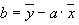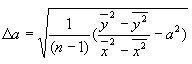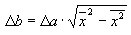If b parameter is equal to zero, then:
y = a*x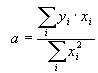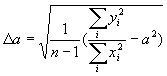Such a method can be applied to any functional dependances. For example, the following problem evolved while discovering the properties of fractal structures (see Percolation theory). Results of experiments are boolean values Resi, which depends on some real number qi. The problem is to determine such critical qcr, that for most qi<qcr results are true, and for most qi>qcr results are false. To find the solution I considered an error function Ri(Resi,qi,qcr):
Ri(Resi,qi,qcr) = 1, if qi<qcr and Resi=False or qi>qcr and Resi=True
Ri(Resi,qi,qcr) = 0 in all other cases.
The error for some qcr is:
E =SRi(Resi,qi,qcr)(qi-qcr)2
To minimize it, we will consider Ri(Resi,qi,qcr) as a constant expression and use some number as initial value for qcr. Differentiating the total error function E over qcr, we can find first approximation for qcr. It is recurrent process, and j-s approximation for qcr is:
qcr j =SRi(Resi,qi,qcr j-1)*qi / SRi(Resi,qi,qcr j-1)
After some iterations an uncertainity "0 / 0" can occur. It is the sign that the iterations must be stopped, because the right result has been already achieved.

Program sources for general case and for case of direct proportional dependance  LSM.pas LSM1.pas
Compiled programs LSM.exe LSM1.exe

#### Calcualtion of implicit functions, specified by define integrals

Functions are often defined implicitly by integrals:
g(a) = тb1b2 f(x,a)dx
Here both b1 and b2 can tend to infinity, or the function f(x,a) itself can tend to infinity at some points between b1 and b2. Approximate calculation of define integral is not the fastest way to calculate the implicit function. Moreover, this way is often useless when the limits of integral b1 and b2 tend to infinity. So what is to be done? Using series expansion and integration of all the terms in the series analytically is quite a convenient method. The simplest possible series are Tailor's series or Fourier series. But there is a small disadvantage: Tailor's series can not be used for integration when the limits of integral tend to infinity. But remember that negative degrees of x or any other function, which is integrable at infinity, can be also used for series expansion. That is:
f(x) = a2/x2 + a3/x3 ... or:
f(x) = a1e-x + a2e-2x ...
and so on. Coefficients ai can be obtained as for Tailor's series:
ai = 1/i! dif(x)/df0i
where f0 is the basic function for series expansion. Coefficients can be also obtained approximately, using Less Square Method, for example. Now it is very simple to perform integration:
тҐa f(x)dx = тҐa a2/x2 + тҐa a3/x3 + ...  = -a2/a - 0.5*a3/a2...
You just have to summarize all the terms of obtained series with required precision. It is very important that series expansion in terms of negative degrees of x should start from x-2 - it enables us to integrate.
Beta-function and gamma-function are important implicit functions.
Beta-function is defined as:
B(p,q) = т10xp-1(1-x)q-1 dx (p>0, q>0)
Gamma-function is defined as:
G(s) = тҐ0xs-1*Exp(-x) dx (s>0)
The procedure which returns coefficients for Tailor series of (1-x)q in terms of x and the procedure calculating beta-function, which uses Tailor series for integration, were included in unit Matrix.pas.

©2002-2003, Veter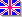English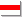Беларуская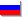Русский
Сайт создан в системе uCoz